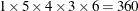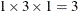### PARAMETERS Statement

PARAMETERS <parameter-specification> <,, parameter-specification>
</ PDATA=SAS-data-set> ;

PARMS <parameter-specification> <,, parameter-specification>
</ PDATA=SAS-data-set> ;

A PARAMETERS (or PARMS) statement is required. The purpose of this statement is to provide starting values for the NLIN procedure. You can provide values that define a point in the parameter space or a set of points.

All parameters must be assigned starting values through the PARAMETERS statement. The NLIN procedure does not assign default starting values to parameters in your model that do not appear in the PARAMETERS statement. However, it is not necessary to supply parameters and starting values explicitly through a parameter-specification. Starting values can also be provided through a data set. The names assigned to parameters must be valid SAS names and must not coincide with names of variables in the input data set (see the DATA= option in the PROC NLIN statement). Parameters that are assigned starting values through the PARAMETERS statement can be omitted from the estimation, for example, if the expression in the MODEL statement does not depend on them.

#### Assigning Starting Values with Parameter-Specification

A parameter-specification has the general form

name = value-list

where name identifies the parameter and value-list provides the set of starting values for the parameter.

Very often, the value-list contains only a single value, but more general and flexible list specifications are possible:

m

a single value

m1, m2, …,several values

m TO n

a sequence where m equals the starting value, n equals the ending value, and the increment equals 1

m TO n BY i

a sequence where m equals the starting value, n equals the ending value, and the increment is i

m1, m2 TO m3

mixed values and sequences

When you specify more than one value for a parameter, PROC NLIN sorts the values in ascending sequence and removes duplicate values from the parameter list before forming the grid for the parameter search. If you specify several values for each parameter, PROC NLIN evaluates the model at each point on the grid. The iterations then commence from the point on the grid that yields the smallest objective function value.

For example, the following PARMS statement specifies five parameters and sets their possible starting values as shown in the table:

parms  b0 = 0
b1 = 4 to 8
b2 = 0 to .6 by .2
b3 = 1, 10, 100
b4 = 0, .5, 1 to 4;


Table 63.5: continued

Possible starting values

B0

B1

B2

B3

B4

0

4

0.0

1

0.0

5

0.2

10

0.5

6

0.4

100

1.0

7

0.6

2.0

8

3.0

4.0

Residual sums of squares are calculated for each of thecombinations of possible starting values. (Specifying a large grid of values can take considerable computing time.)

If you specify a starting value with a parameter-specification, any starting values provided for this parameter through the PDATA= data set are ignored. The parameter-specification overrides the information in the PDATA= data set. When you specify a BY statement, the same parameter-specification is applied in each BY group. To vary starting values with BY groups, use the PDATA= option in the PARAMETERS statement as described in the following paragraphs.

#### Assigning Starting Values from a SAS Data Set

The PDATA= option in the PARAMETERS statement enables you to assign starting values for parameters through a SAS data set. The data set must contain at least two variables that identify the parameter and contain starting values, respectively. The character variable identifying the parameters must be named Parameter or Parm. The numeric variable containing the starting value must be named Estimate or Est. This enables you, for example, to use the contents of the ParameterEstimates table from one PROC NLIN run to supply starting values for a subsequent run, as in the following example:

proc nlin data=FirstData;
parameters alpha=100 beta=3 gamma=4;
< other NLIN statements >
model y = ... ;
ods output ParameterEstimates=pest;
run;

proc nlin data=SecondData;
parameters / pdata=pest;
Switch = 1/(1+gamma*exp(beta*log(dose)));
model y = alpha*Switch;
run;


You can specify multiple values for a parameter in the PDATA= data set, and the parameters can appear in any order. The starting values are collected by parameter and arranged in ascending order, and duplicate values are removed. The parameter names in the PDATA= data set are not case sensitive. For example, the following DATA step defines starting values for three parameters and a starting grid withpoints:

data test;
input Parameter \$ Estimate;
datalines;
alpha  100
BETA   4
beta   4.1
beta    4.2
beta    4.1
gamma 30
;


If starting values for a parameter are provided through the PDATA= data set and through an explicit parameter-specification, the latter takes precedence.

When you specify a BY statement, you can control whether the same starting values are applied to each BY group or whether the starting values are varied. If the BY variables are not present in the PDATA= data set, the entire contents of the PDATA= data set are applied in each BY group. If the BY variables are present in the PDATA= data set, then BY-group-specific starting values are assigned.